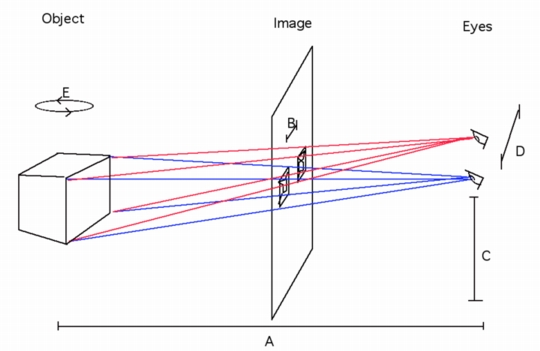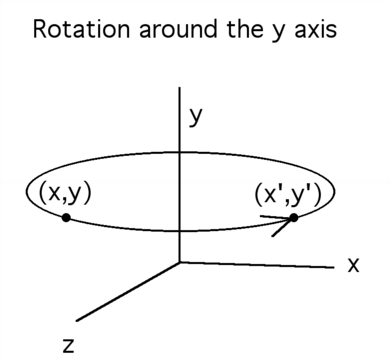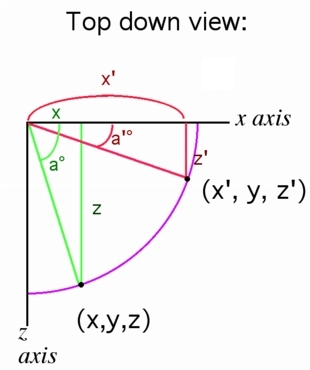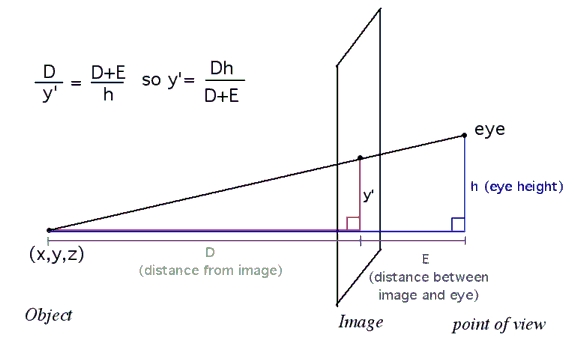#### Stereoscopic Dots

Drag to rotate around the vertical axis

Your browser does not support HTML5 canvas.## The Rotation & Perspective FormulasAs the point revolves around the y axis, it follows the path of a circle, always at the same height(y) and distance (the radius of the circle) from the turning point (the y axis). A green right triangle can be formed from the turning point, the original location (x,y,z) and the axes. Since sin a°= opposite/hypotenuse, then sin a° = z/d, where d is the distance of the point to the turning point (or the radius of the circle). Solving the equation for z, z= d * sin a° Therefore, to get the new location (x', y, z') we can see the red triangle can be formed, and z' = d * sin a'° By similar means the new x' will be x' = d cos a'°, Since cos a° = adjacent/hypotenuse and in the green triangle cos a° = x/d so that x = d * cos a°The adjustment for perspective takes advantage of the similar triangles that are formed between the object, the eye and the image in between. The diagram above shows only how to find the vertical adjustment y' (the corresponding vertical coordinate on the image would be y+y'), but the horizontal coordinate is found in exactly the same way. The ratios between the similar triangles are the same, and so solving for y', it is found to be the ratio between the product of the Distance and observer's height and the total distance between the object and the observer.

## What's next?

The wonderful brain you have helps you to see two images as one, with an intuitive sense of the geometry of two view points. The closer the object the more different the view on the left is from the view on the right. Another explanation is here